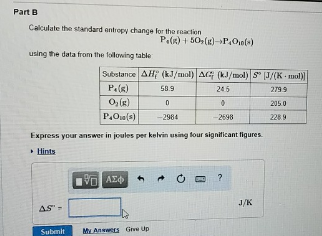Chemistry Practice Problems Entropy Practice Problems Solution: Part BCalculate the standard entropy change for th...

🤓 Based on our data, we think this question is relevant for Professor Mack's class at CSUS.

# Solution: Part BCalculate the standard entropy change for the reaction P4 (g) + 5O2 (g) → P4O10 (s) using the data from the following table: Express your answer in joules per kelvin using four significant figures.

###### Problem

Part B

Calculate the standard entropy change for the reaction

P4 (g) + 5O2 (g) → P4O10 (s)

using the data from the following table:View Complete Written Solution

Entropy

Entropy

#### Q. Entropy of Reaction for Nitrogen Dioxide Formation Nitric oxides. NO and NO2, contribute to air pollution, acid rain, and the depletion of the ozone l...

Solved • Mon Sep 03 2018 13:20:25 GMT-0400 (EDT)

Entropy

#### Q. Calculate the standard entropy change for the following reaction at 25°C. S° values can be found here. Mg(OH)2 (s) + 2HCl (g) → MgCl2 (s) + 2H2O (g)

Solved • Wed Aug 29 2018 12:43:31 GMT-0400 (EDT)

Entropy

#### Q. The ΔHvap of a certain compound is 28.26 kJ mol and its ΔSvap is 50.01 J•mol-1•K-1. What is the boiling point of this compound?

Solved • Tue Aug 28 2018 12:51:36 GMT-0400 (EDT)

Entropy

#### Q. Calculate the standard entropy change for the following reaction, 2SO2 (g) + O2 (g) → 2SO3 (g) given S° [SO2 (g)] = 248.2 J/K • mol. S° [O2 (g)] = 205...

Solved • Mon Aug 27 2018 15:20:27 GMT-0400 (EDT)